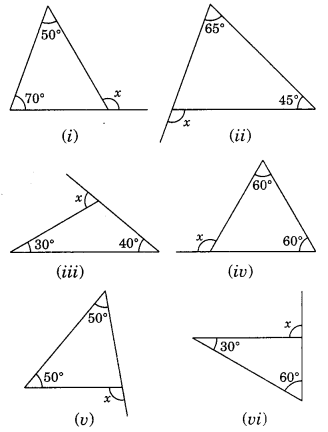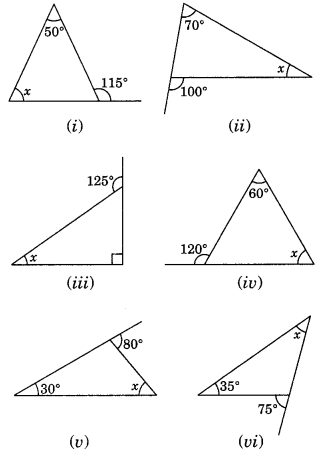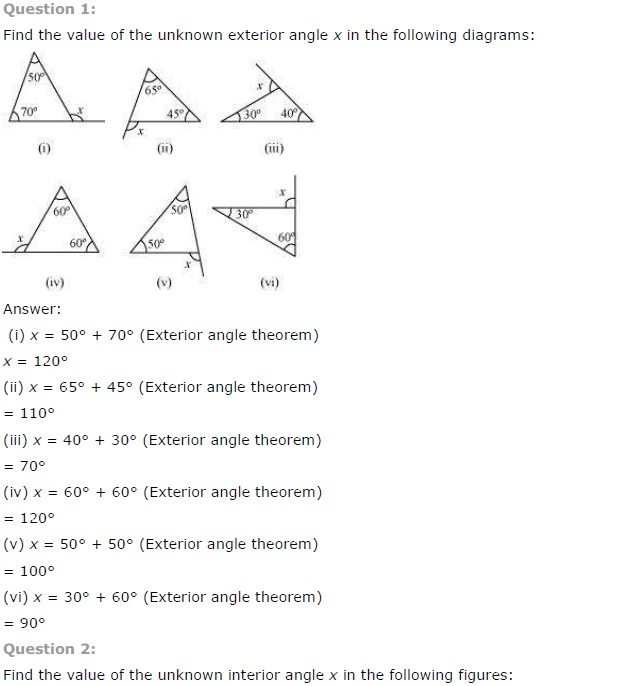# NCERT Solutions for Class 7 Maths Chapter 6 The Triangle and its Properties Ex 6.2

NCERT Solutions for Class 7 Maths Chapter 6 The Triangle and its Properties Ex 6.2

### NCERT Solutions for Class 7 Maths Chapter 6 The Triangle and its Properties Ex 6.2

NCERT Solutions for Class 7 Maths Chapter 6 The Triangle and its Properties Exercise 6.2
Ex 6.2 Class 7 Maths Question 1.
Find the value of the unknown exterior angle x in the following diagrams:Solution:
(i) ∠x = 50° + 70° = 120° (Exterior angle is equal to sum of its interior opposite angles)
(ii) ∠x = 65°+ 45° = 110° (Exterior angle is equal to sum of its interior opposite angles)
(iii) ∠x = 30° + 40° = 70° (Exterior angle is equal to sum of its interior opposite angles)
(iv) ∠x = 60° + 60° = 120° (Exterior angle is equal to sum of its interior opposite angles)
(v) ∠x = 50° + 50° =100° (Exterior angle is equal to sum of its interior opposite angles)
(vi) ∠x = 30° + 60° = 90° (Exterior angle is equal to sum of its interior opposite angle)

Ex 6.2 Class 7 Maths Question 2.
Find the value of the unknown interior angle x in the following figures:Solution:
(i) ∠x + 50° = 115° (Exterior angle of a triangle)
∴ ∠x = 115°- 50° = 65°
(ii) ∠x + 70° = 110° (Exterior angle of a triangle)
∴ ∠x = 110° – 70° = 40°
(iii) ∠ x + 90° = 125° (Exterior angle of a right triangle)
∴ ∠x = 125° – 90° = 35°
(iv) ∠x + 60° = 120° (Exterior angle of a triangle)
∴ ∠x = 120° – 60° = 60°
(v)∠ X + 30° = 80° (Exterior angle of a triangle)
∴ ∠x = 80° – 30° = 50°
(vi) ∠ x + 35° = 75° (Exterior angle of a triangle)
∴ ∠ x = 75° – 35° = 40°## SabDekho

The Complete Educational Website